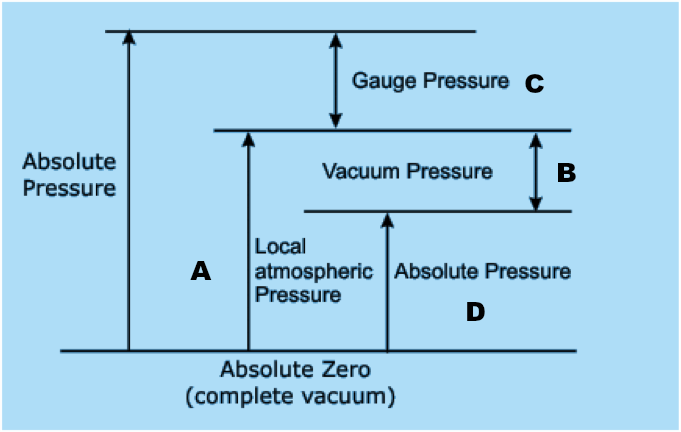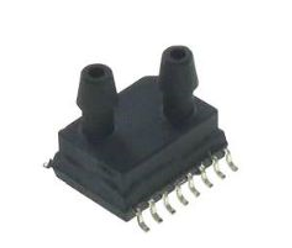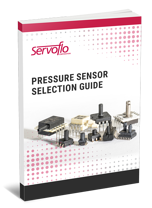There are two types of pressure sensors used for negative pressure or vacuum pressure applications: gauge sensors or absolute pressure sensors. The reference pressure is the differentiating factor between these two types of sensors. The diagram below illustrates how the different methodologies work.Figure 1: The diagram illustrates how the different methodologies work. Source: Servoflo

In applications that rely on the principle of a vacuum, it is important to effectively measure negative pressure (also known as vacuum pressure). There are two basic ways to do so:

• Measure the amount of pressure below local atmospheric pressure (typically 14.7 pounds/square inch absolute (PSIA))
• Measure how much pressure is above absolute zero vacuum (0 PSIA)

Both methods of measurement utilize the same scale and pressure point. However, these two measurements will yield differing results over time because one measurement's reference point is a fixed quantity (absolute zero vacuum), whereas the other relies upon a variable amount (atmospheric air pressure, which can fluctuate).

1. In the case of a gauge sensor, either a single port or dual port model can be used. In the case of a dual port gauge sensor, the "lower pressure" port measures the negative or vacuum pressure.
2. By accessing the "lower pressure" port, the user is applying a positive pressure to the higher pressure port as in pressure range.
3. The advantage of using a gauge sensor is if the user wants to measure a vacuum smaller than the full vacuum rangeFigure 2: By using a gauge sensor, the user can capitalize on higher resolution and measure smaller negative pressure ranges. Source: Servoflo14.7 PSI, such as -3 PSI, using a lower range focusing on the negative pressure range is more appropriate. This way, by using a smaller full-scale range pressure sensor, the user captures the full-range of the vacuum pressure measurements, thus obtaining more resolution and more design flexibility. For a single port gauge sensor, the single port is vented to atmosphere.

For example, if a user is trying to measure the inhalation pressure in a respiratory application, the pressure range would be no more than 0.6 PSI. If an absolute pressure sensor is used, only 4% of the pressure range would be used, thereby wasting 96% of the sensor's calibrated range. By using a gauge sensor such as the AL4 Series, the user can capitalize on higher resolution and measure smaller negative pressure ranges.

## Measuring pressure below local atmospheric pressure sources

Vacuum pressure is typically measured in terms of PSI or pounds per square inch vacuum (PSIV). This measurement is generally relative to the surrounding atmospheric pressure. Vacuum pressure transducers can convert the inner negative pressure contained by an application into an electrical signal, and from there into a precise measurement.

At 0 PSIV — which is the same as typical atmospheric pressure, or 14.7 PSIA — the electrical output of a vacuum pressure transducer is 0 V DC. At full-scale vacuum (14.7 PSIV or 0 PSIA), the output generally increased to about 5 V DC. A vacuum pressure transducer's output in volts increases proportionally to the increase in vacuum or negative pressure. On the other hand, an absolute pressure transducer emits increased positive voltage in proportion to decreasing vacuum.

## Measuring how much pressure is above absolute zero vacuum sources

The scale for absolute pressure begins at the high vacuum point of 0 PSIA. Unlike measurements that rely on atmospheric pressure as their main reference point, this methodology allows for more consistent readings.

An absolute pressure transducer features an electrical output of 0 V DC at 0 PSIA and increases to 5 V DC when it reaches full-scale pressure (14.7 PSIA). This output is expressed in positive voltage.

## Pressure sensors that measure negative pressure

Vacuum pressure transducers and absolute pressure transducers measure the same thing using different points of reference. They are both crucial components for use in applications that require accurate and reliable negative pressure measurements for proper functionality and optimal performance.

When attempting to measure negative air pressure, it is important to use the proper equipment and to have that equipment calibrated to yield correct readings. Even small discrepancies between measurement and actual vacuum level could lead to performance issues.

Examples of classic applications where negative pressure measurements are needed include negative wound pressure therapy, breathing applications, pump control/performance monitoring, HVAC applications such as filter monitoring and pressurized rooms such as cleanrooms and isolation rooms. One pressure sensor series that works well for these applications is the AP/AG Series from Fujikura.Figure 3: The Pressure Sensor Selection Guide. Source: ServofloAbout Servoflo